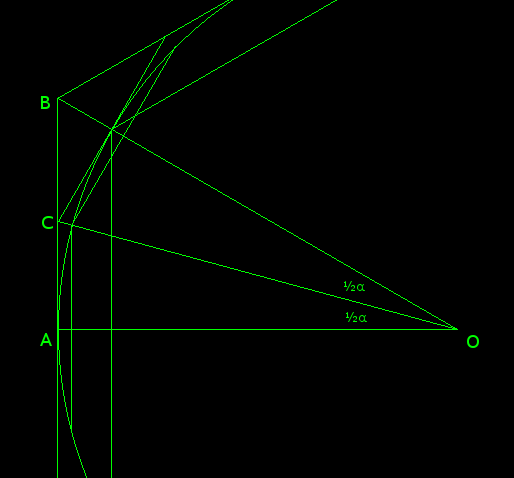## 14-Mar-2008

### Archimedes simplified

Filed under: math — jlm @ 12:15

In the third century BC, Archimedes calculated an amazing approximation to π, bettering the old value of 256/81 worked out by 17th century Egyptians.
How did he do it? He circumscribed and inscribed a hexagon around a circle, then bisected each hexagon’s central angles to make a dodecagon, then a 24-gon, 48-gon, and finally a 96-gon. The perimeters of the polygons bound π above and below, and each pair of perimeters is related to the ones before.
Consider a pair of polygons being bisected:If the radius AO = 1, then AB = tan α and AC = tan ½α.
If it has n sides, the outer perimeter P = 2n tan α and the new polygon has perimeter P’ = 4n tan ½α. The inner perimeter is p = 2n sin α, with the new polygon’s perimeter being p’ = 4n sin ½α.

Now, 1/P + 1/p = 1/(2n tan α) + 1/(2n sin α) = (sin α + tan α)/(2n sin α tan α) = (1 + sec α)/(2n tan α) = (cos α + 1)/(2n sin α) = (cos² ½α – sin² ½α + 1)/(4n sin ½α cos ½α) = (2 cos² ½α – 1 + 1)/(4n sin ½α cos ½α) = (1/2n) cot ½α = 2/P’
And, P’ p = (4n tan ½α)(2n sin α) = 16n² tan ½α sin ½α cos ½α = 16n² sin² ½α = p’²
So, we have easy ways to calculate successive perimeters from our basic hexagons, with p = 6, P = 4 √3, using only simple arithmetic and square roots.

n P p
6 6.928203 6
12 6.430781 6.211657
24 6.319320 6.265257
48 6.292172 6.278700
96 6.285429 6.282064

which puts 3.1427 > π > 3.1410.

Now, Archimedes didn’t have trigonometric functions to utilize in his calculations, so he calculated the perimeters using similar triangles, a much more complicated process. But it gives the same values. He also lacked decimals, so he worked with rationals instead, producing the bounds 3 1/7 > π > 3 10/71.